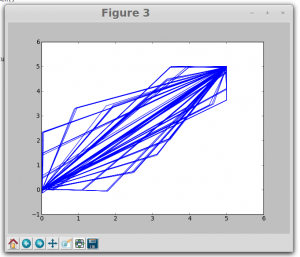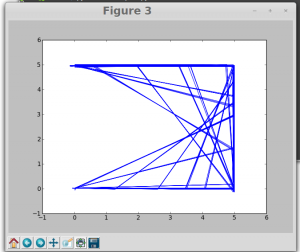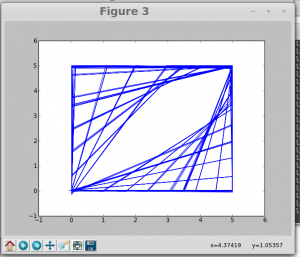# Sunday, 22nd June 2014

Continued to work on experiments to produce Lissajous Figures. Generated two square waves with a phase co relation between  them. Used Capture2 function to capture data and plot graphs. The figures are sensitive to phase difference and the ratio of frequencies.

This is the code used.

from pylab import *
import expeyes.eyesj
p = expeyes.eyesj.open()

#p.set_sqr1(8000)
#p.set_sqr2(4000)
p.set_sqrs(8000,13)

t1,v1,t2,v2 = p.capture2(1, 2, 400, 16)
figure(3)
plot(v1,v2)
show()

These are the patterns obtained…The smooth Lissajous Figures are expected if we use two sine waves instead of square waves. Working on the module to generate two sine  waves of variable frequency.

Thats all for today…….:)